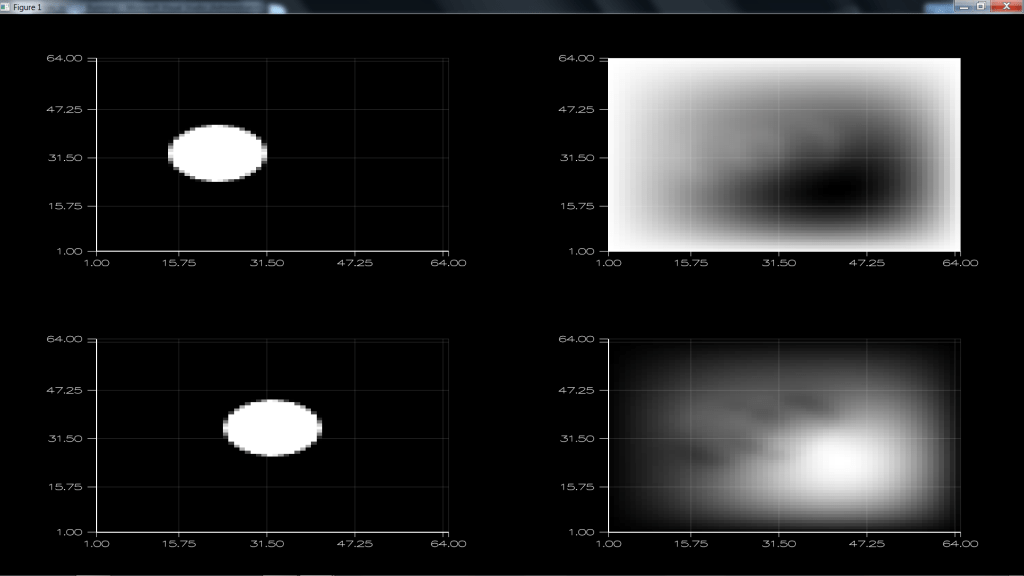# ArrayFire Examples (Part 4 of 8) – Image Processing

This is the fourth in a series of posts looking at our current ArrayFire examples. The code can be compiled and run from `arrayfire/examples/` when you download and install the ArrayFire library. Today we will discuss the examples found in the `image_processing/` directory.

In these examples, my machine has the following configuration:

```ArrayFire v1.9 (build XXXXXXX) by AccelerEyes (64-bit Windows)
CUDA toolkit 5.0, driver 306.94
GPU0 GeForce GT 650M, 2048 MB, Compute 3.0 (single,double)
Display Device: GPU0 GeForce GT 650M
Memory Usage: 1981 MB free (2048 MB total)...```

Image Demo

The purpose of this example is to show how to do some common image manipulations. The method `channel_split` shows how easily multi-dimensional arrays can be subdivided:

```// Split a MxNx3 image into 3 separate channel matrices.
static void channel_split(array& rgb, array& outr, array& outg, array& outb) {
outr = rgb(span, span, 0);
outg = rgb(span, span, 1);
outb = rgb(span, span, 2);
}```

The colorspace function is useful for moving images between representation spaces. Images can easily be loaded as color images, or converted to grayscale images. Note that when loading images, scaler division is performed to normalize the values between 0 and 1:

`array img_rgb  = loadimage("logo.jpg", true) / 255.f; // 3 channel RGB       [0-1]`

Other useful functions in this example include computing the histogram, equalization, and calculating thresholds:

```    // connected component labeling
array B = img_gray < 190; // binary [0 1]```

This is just a small sample of what our library can do, check out all of our image processing functions here.

Running the example produces the following output:Optical Flow

Optical Flow is the apparent motion of an object due to the difference in motion between the object and its observer. Horn-Schunck is a mathematical method for calculating optical flow using integration techniques. As usual for these examples, it isn’t so much about understanding the math, as it is seeing the results of ArrayFire applied to the math. What this example does essentially is convolve images repeatedly for a period of time. Being able to convolve these images on the GPU greatly increases the speed with which we can simulate the integration. Writing the code is simple with ArrayFire, we simply encode the mathematics in a way that is simple to follow:

```        array u_ = convolve(u, mean_kernel);
array v_ = convolve(v, mean_kernel);

const float alphasq = 0.1f;
array num = Ix * u_ + Iy * v_ + It;
array den = alphasq + Ix * Ix + Iy * Iy;

array tmp = 0.01 * num;
u = u_ - (Ix * tmp) / den;
v = v_ - (Iy * tmp) / den;```

This example is best run on your own computer so you can see the results in action, here is what the final output looks like:Being able to repeat image calculations for a period of time is a technique for solving a number of important image processing problems. The GPU is perfect for these kinds of algorithms, because each operation can be performed to an entire image at once (like a convolution). This allows us to get results that are much more real time.

What other image processing applications can you see for GPU processing and ArrayFire? Download the ArrayFire library and find out today!

Posts in this series: